#Quality Digest

Featured Product
This Week in Quality Digest Live
Six Sigma Features
William A. Levinson
Quality and manufacturing professionals are in the best position to eradicate inflationary waste
Donald J. Wheeler
What does this ratio tell us?
Donald J. Wheeler
How you sample your process matters
Paul Laughlin
How to think differently about data usage
Donald J. Wheeler
The origin of the error function
Six Sigma News
How to use Minitab statistical functions to improve business processes
Sept. 28–29, 2022, at the MassMutual Center in Springfield, MA
Elsmar Cove is a leading forum for quality and standards compliance
Is the future of quality management actually business management?
Too often process enhancements occur in silos where there is little positive impact on the big picture
Collect measurements, visual defect information, simple Go/No-Go situations from any online device
Good quality is adding an average of 11 percent to organizations’ revenue growth
Floor symbols and decals create a SMART floor environment, adding visual organization to any environment
A guide for practitioners and managersBio

Six Sigma

## Skewness Visualized

### What you think you know may not be so

Published: Monday, January 9, 2023 - 13:03

The computation for skewness does not fully describe everything that happens as a distribution becomes more skewed. Here we shall use some examples to visualize just what skewness does—and does not—involve.

The mean for a probability model describes the balance point. The standard deviation describes the dispersion about the mean. Yet a simple description of skewness is elusive. Depending on which book you read, skewness may be described as having something to do with the relative size of the two tails, or with the weight of the heavier tail of a probability model.

By far the easiest way to understand what increasing skewness does to a probability model is to compare models with different amounts of skewness. But before we can do this, we have to first standardize those models. This is because skewness is defined in terms of standardized variables; skewness is what happens after we have taken into account differences in location and dispersion. (If we compare two distributions that have not been standardized, differences in location and dispersion may obscure differences in skewness.) So here we will be working with standardized distributions where the mean is always zero and the standard deviation is always equal to one.

In an earlier column, I demonstrated how the formula for skewness depends upon the extreme values in the heavier tail of a probability model. Since these values will tend to be part of the invisible portion of the probability model, it will be difficult to see the direct effects of increasing skewness. However, in this case the tail does wag the dog, and there are certain indirect results of increasing skewness that are easy to see. It is these visual characteristics that we want to discover.

To isolate the effects of skewness from those of kurtosis, we will need to use a family of distributions where we can change the skewness without simultaneously changing the kurtosis. This requirement rules out many commonly used distributions such as the gammas, Weibulls, and lognormals where the two shape parameters always change together. In what follows, we will use the family of Burr distributions. (Next month’s column will consider the visual effects of increasing kurtosis.)

### Comparison one: Skewness changes from 0.0 to 0.8

The Burr distribution at the top of Figure 1 is very nearly symmetric. It has a skewness of 0.05 and a kurtosis of 3.90. The skewed Burr distribution at the bottom has a skewness of 0.84 and a kurtosis of 3.90. This distribution is near the maximum possible skewness that a mound-shaped distribution can have when the kurtosis is 3.90. So the difference between these two distributions comes close to the maximum possible shift in skewness for this level of kurtosis.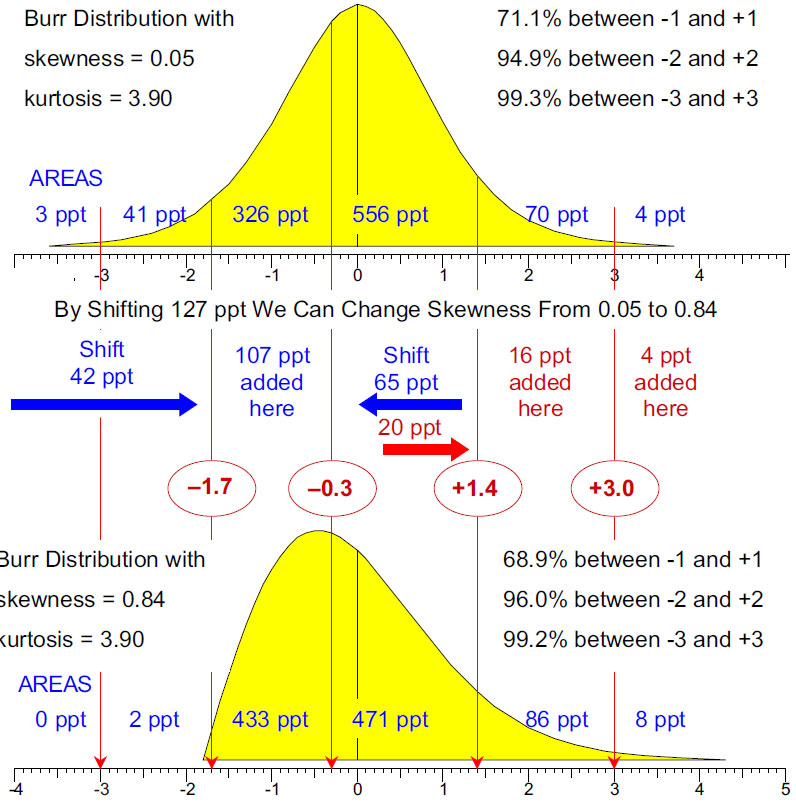Figure 1: Changing skewness from 0.05 to 0.84

To change the first distribution of Figure 1 into the second, a total of 127 parts per thousand need to be shifted around. From the region below -1.7 we will need to shift 42 ppt up into the region between -1.7 and -0.3. From the region between -0.3 and +1.4 we will need to shift 65 ppt down into the region between -1.7 and -0.3. And from this same region between -0.3 and +1.4 we will also need to shift 20 ppt up into the upper tail. Of these 20 ppt, 16 will be added to the region between +1.4 and +3.0, and 4 will be added to the tail out beyond +3.0.

So, of the 127 ppt that were shifted, 107 ppt went into the left central mound and only 20 ppt went into the upper tail. The 107 ppt shifted inward compensate for the increased rotational inertia of the 20 ppt shifted outward. They keep the mean at zero, the standard deviation at one, and the kurtosis at 3.90, while allowing the skewness to change from 0.05 to 0.84

So what is the visual impact of the difference in these two extremes of skewness? While skewness here is a function of the upper tails, in Figure 2 we can only see a small difference in the two upper tails between +1.4 and +3.0. And out beyond +3.0, the differences between these two upper tails disappear.Figure 2: Skewness = 0 and skewness = 0.84 when kurtosis = 3.90

However, below +1.4 there are major shifts as the central mound becomes lopsided and the lower tail disappears. So while 49 percent of the skewness value of 0.84 for the second distribution depends solely on the 8 ppt beyond +3.0, the visible manifestations of skewness are the lopsided mound and a shorter lower tail that come from the 107 ppt shifting into the region between –1.7 and –0.3.

### Comparison two: Skewness changes from 0.8 to 1.4

This comparison will use Burr distributions having a kurtosis of 6.0. Here we shall compare a Burr having a skewness of 0.84 with a Burr having a skewness of 1.42. These are near the possible extremes of skewness for Burr distributions having a kurtosis of 6.0.Figure 3: Changing skewness from 0.84 to 1.42

To change the first distribution of Figure 3 into the second, a total of 157 parts per thousand need to be shifted around. From the region below -1.3 we need to shift 70 ppt up into the region between -1.3 and -0.3. From the region between -0.3 and +1.4 we need to shift 72 ppt down into the region between -1.3 and -0.3. And from this same region between -0.3 and +1.4 we need to shift 15 ppt up into the upper tail above +1.4. Of these 15 ppt, 11 will be added to the region between +1.4 and +3.0 and 4 will be added to the tail out beyond +3.0.

So, of the 157 ppt that were shifted, 142 ppt went into the left central mound and only 15 ppt went into the upper tail. The 142 ppt shifted inward compensate for the increased rotational inertia of the 15 ppt shifted outward. They keep the mean at zero, the standard deviation at 1, and the kurtosis at 6.00, while allowing the skewness to change from 0.84 to 1.42

So what is the visual impact of the difference in these two extremes of skewness? While skewness here is a function of the upper tails, in Figure 4 we can only see a small difference in the two upper tails between +1.4 and +3.0, and out beyond +3.0 the differences between these two tails disappear.Figure 4: Skewness = 0.84 and skewness = 1.42 when kurtosis = 6.0

However, below +1.4 there are major shifts as the central mound becomes lopsided and the lower tail disappears. So while 62 percent of the skewness value of 1.42 for the second distribution depends solely on the 14 ppt beyond +3.0, the visible manifestations of increasing skewness are the lopsided mound and truncated lower tail that come from the 142 ppt shifting into the region between –1.3 and –0.3.

### Comparison three: Skewness changes from 1.4 to 2.1

This comparison will use Burr distributions having a kurtosis of 10.0. Here we shall compare a Burr having a skewness of 1.41 with a Burr having a skewness of 2.12. Once again these are near the possible extremes of skewness for Burr distributions having a kurtosis of 10.0.Figure 5: Changing skewness from 1.41 to 2.12

To change the first distribution in Figure 5 into the second, a total of 230 parts per thousand need to be shifted around. From the region below -1.0, we need to shift 127 ppt up into the region between -1.0 and -0.3. From the region between -0.3 and +1.8 we need to shift 90 ppt down into the region between -1.0 and -0.3. And from this same region between -0.3 and +1.8 we need to shift 13 ppt up into the upper tail above +1.8. Of these 13 ppt, 6 will be added to the region between +1.8 and +3.0 and 7 will be added to the tail out beyond +3.0.

So, of the 230 ppt that were shifted, 217 ppt went into the left central mound and only 13 ppt went into the upper tail. The 217 ppt shifted inward compensate for the increased rotational inertia of the 13 ppt shifted outward. They keep the mean at zero, the standard deviation at 1, and the kurtosis at 10.0, while allowing the skewness to change from 1.41 to 2.12

So what is the visual impact of the difference in these two extremes of skewness? While skewness here is a function of the upper tails, in Figure 6 we can barely see any difference between the two upper tails.Figure 6: Skewness = 1.41 and skewness = 2.12 when kurtosis = 10.0

However, below +1.8 there are major shifts as the central mound becomes lopsided and the lower tail disappears. So while 73 percent of the skewness value of 2.12 for the second distribution depends solely on the 18 ppt beyond +3.0, the visible manifestations of increasing skewness are the lopsided mound and missing lower tail that come from the 217 ppt shifting into the region between –1.0 and –0.3.

### Why don’t the tails get heavier?

With these three comparisons we have chased the skewness from 0.05 to 2.12. Each comparison effectively shows the maximum amount by which the skewness can change at that step. These maximal changes in skewness show that the upper tails do get heavier with increasing skewness, but only by small amounts. Why don’t the upper tails show greater increases in weight? Why does skewness work this way?

The answer lies in the basic calculus of probability theory. In order for any probability model to have values for skewness and kurtosis, the standardized probability density function, f(z), must go to zero faster than the fourth power of z goes to infinity—otherwise the integrals would not converge to a limiting value. As a consequence, there are limits on how much weight can be placed in the tails of any distribution.

### Areas within two and three sigma

The variance of a probability model is simply another name for rotational inertia about the mean. And you cannot cheat on rotational inertia. It imposes certain requirements on all probability models. The requirements of interest here are those imposed on mound-shaped (unimodal) probability models.

Figures 1, 3, and 5 show one of these requirements. Mound-shaped distributions that are typically used to model data will have between 94.9 percent and 96.4 percent of their area within two standard deviations of the mean. Consequently, only three to four percent of the area is available for skewness to move into the tails beyond two sigma, which explains why the upper tails grow so slowly with increasing skewness.

Furthermore, regardless of the skewness or kurtosis, a mound-shaped probability model such as those used here will always have at least 98 percent of its area within three standard deviations of the mean.

### Summary

So what can we learn from these comparisons? While skewness is a mathematical function of the heavier tail of a distribution, the areas involved are very small, amounting to a few parts per thousand with even the most extreme increases in skewness. As seen in Figure 7, the differences between the upper tails are minuscule. The visible effects of increasing skewness are the shortening of one tail and a lopsided central mound on the side with the short tail.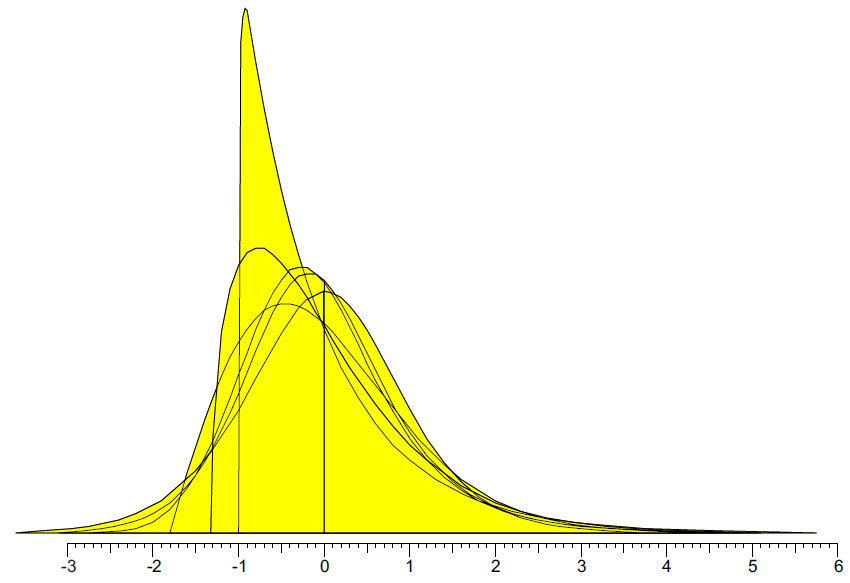Figure 7: The effects of increasing skewness from 0.05 to 2.12

So the mathematical function we call skewness is an indirect measure of the actual changes in shape for the distribution. Data coming from a process with a skewed distribution will always display the twin visual effects but will have little to show in the elongated tail. So, contrary to popular opinion, increasing positive skewness does not mean that the upper tail gets appreciably heavier or noticeably elongated. These increases are measured in parts per thousand rather than parts per hundred.

This means that a bell-shaped histogram with a few outliers on one side is not likely to represent a process having a skewed distribution. When analyzing data, we need to be careful about this point. Most “skewed” histograms encountered in practice result from smash-ups of data collected while the process was changing Such histograms may have a long tail, but they are unlikely to be a representation of a skewed probability model. So do not make the mistake of interpreting outlying points as evidence of skewness.

How can you identify outlying points? The easiest, most robust way of detecting outliers is to use a process behavior chart with its generic, fixed-width, three-sigma limits. When used with rational subgrouping and rational sampling it is equal to, or better than, all other outlier detection techniques. Once we have an understanding of what skewness does, and what it does not do to a probability distribution, we can begin to see why a process behavior chart works regardless of the shape of our histogram.

### Appendix: Burr distributions

The interested reader can verify all of the results above using the following. In 1972, Irving Burr discovered and investigated a family of probability models for x>0 having a cumulative distribution function of the form:The probability density function is: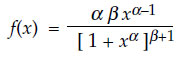where α>0 and ß>0 are the parameters of the Burr distribution.

In order for a Burr distribution to have a well-defined mean, standard deviation, skewness, and kurtosis, the product of the two parameters must exceed 4.0, that is:
α ß > 4.0

When this is the case, the mean, standard deviation, skewness, and kurtosis are defined by the following formulas:and where the symbol Γ(α) denotes the gamma func­tion (for α>0):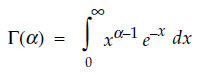### Standardized Burr distributions

If we let z denote the value on the horizontal axis for a standardized Burr distribution, the cumulative standardized Burr distribution function will have the form: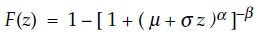where z>–µ/σ and µ and σ are the mean and standard deviation defined above for the Burr distribution with parameters α and ß. This allows for the easy computation of the probability that a value is less than some specific z-score.

The standardized probability density function is:This will facilitate drawing the density function for a given standardized Burr distribution. The six Burr distributions used here are listed in the table in Figure 8.Figure 8: Burr distributions used

### Discuss### Donald J. Wheeler

Dr. Wheeler is a fellow of both the American Statistical Association and the American Society for Quality who has taught more than 1,000 seminars in 17 countries on six continents. He welcomes your questions; you can contact him at djwheeler@spcpress.com.

### Process Behavior Charts are easy

What will be upsetting for all those folk who did courses in the Six Sigma Scam and were told to buy expensive statistical software to account for skewness is that you have wasted your time and money.

Process Behavior Charts are easy for all employees.  You can draw them manually.

### Clarity!

Don,

Of the many articles you have written over the years on this subject, this was probably the clearest explanation yet. To those who insisted on disregarding the empirical evidence shown in your work (e.g., Normality and the Process Behavior Chart), this will hopefully provide a clear and technical enough explanation that should be more problematic to refute.

You should consider a new edition of Normality to include some of this recent work.

Congratulations!

Best regards,

Rip